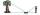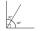# Ruler

How far from Peter stands 2m hight John? Petr is looking to John over ruler that keeps at arm's distant 60 cm from the eye and on the ruler John measured the height of 15 mm.

Result

x =  80 m

#### Solution:

x / 2 = 0.60 / 0.015

x = 80

x = 80

Calculated by our simple equation calculator.

Leave us a comment of example and its solution (i.e. if it is still somewhat unclear...):

Showing 0 comments:Be the first to comment!#### To solve this example are needed these knowledge from mathematics:

Do you have a linear equation or system of equations and looking for its solution? Or do you have quadratic equation? Do you want to convert length units? See also our right triangle calculator. See also our trigonometric triangle calculator. Try conversion angle units angle degrees, minutes, seconds, radians, grads.

## Next similar examples:

1. A boyA boy of height 1.7m is standing 30m away from flag staff on the same level ground . He observes that the angle of deviation of the top of flag staff is 30 degree. Calculate the height of flag staff.
2. Wood dividingWhich equation calculates the number of 1/3-foot pieces that can be cut from a piece of wood that is 7 feet long?
3. Spruce heightHow tall was spruce that was cut at an altitude of 8m above the ground and the top landed at a distance of 15m from the heel of the tree?
4. ThalesThales is 1 m from the hole. The eyes are 150 cm above the ground and look into the hole with a diameter of 120 cm as shown. Calculate the depth of the hole.
5. MirrorHow far must Paul place a mirror to see the top of the tower 12 m high? The height of Paul's eyes above the horizontal plane is 160 cm and Paul is from the tower distant 20 m.
6. Tree shadowTree perpendicular to the horizontal surface has a shadow 8.32 meters long. At the same time meter rod perpendicular to the horizontal surface has shadow 64 cm long. How tall is tree?
7. Mast shadowMast has 13 m long shadow on a slope rising from the mast foot in the direction of the shadow angle at angle 15°. Determine the height of the mast, if the sun above the horizon is at angle 33°. Use the law of sines.
8. Shadow of treeMiro stands under a tree and watching its shadow and shadow of the tree. Miro is 180 cm tall and its shade is 1.5 m long. The shadow of the tree is three times as long as Miro's shadow. How tall is the tree in meters?
9. Cable carCable car rises at an angle 45° and connects the upper and lower station with an altitude difference of 744 m. How long is "endless" tow rope?
10. Complementary angles 2Two complementary angles are (x+4) and (2x - 7) find the value of x
11. Triangle P2Can triangle have two right angles?
12. AnglesIn the triangle ABC, the ratio of angles is: a:b = 4: 5. The angle c is 36°. How big are the angles a, b?
13. SimilarityAre two right triangles similar to each other if the first one has a acute angle 70° and second one has acute angle 20°?
14. Two anglesThe triangles ABC and A'B'C 'are similar. In the ABC triangle, the two angles are 25° and 65°. Explain why in the triangle A'B'C 'is the sum of two angles of 90 degrees.
15. Largest angle of the triangleWhat is the largest angle of the triangle if the second angle is 10° greater than twice the first and the third is 30° smaller than the second?
16. Find the 9Find the missing angle in the triangle and then name triangle. Angles are: 95, 2x+15, x+3
17. If theIf the tangent of an angle of a right angled triangle is 0.8. Then its longest side is. .. .